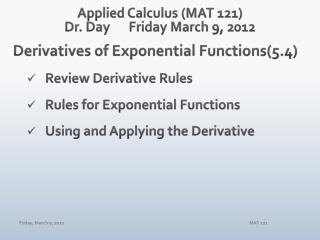# Applied Calculus (MAT 121) Dr. Day Friday March 9, 2012 - PowerPoint PPT PresentationDownload PresentationApplied Calculus (MAT 121) Dr. Day Friday March 9, 2012

Applied Calculus (MAT 121) Dr. Day Friday March 9, 2012Download Presentation## Applied Calculus (MAT 121) Dr. Day Friday March 9, 2012

- - - - - - - - - - - - - - - - - - - - - - - - - - - E N D - - - - - - - - - - - - - - - - - - - - - - - - - - -
##### Presentation Transcript

1. Applied Calculus (MAT 121)Dr. Day Friday March 9, 2012 Derivatives of Exponential Functions(5.4) • Review Derivative Rules • Rules for Exponential Functions • Using and Applying the Derivative MAT 121

2. Exponential Functions Big Ideas • What are Exponential Growth and Exponential Decay? • What sort of applications use exponential functions as their mathematical models? • What algebra do we need to remember to work with exponential functions? • How do we undo exponentiation? We need the inverse function, called the logarithm function. • Where does calculus fit in to all of this? MAT 121

3. Using Logarithms to Solve Equations • Halley's law states that the barometric pressure (in inches of mercury) at an altitude of x mi above sea level is modeled by the function p(x) = 29.92e-0.2x   (x≥ 0) If the barometric pressure as measured by a hot-air balloonist is 23.2 in. of mercury, what is the balloonist's altitude? Round your answer to two decimal places. MAT 121

4. Compound Interest Periodic Compounding • A: amount in account • P: amount of original deposit (principal) • i: annual interest rate, expresses as a decimal value • n: number of compounding periods per year • t: number of years the principal remains in account Continuous Compounding • A: amount in account • P: amount of original deposit (principal) • r: annual interest rate, expresses as a decimal value • t: number of years the principal remains in account MAT 121

5. Review: Derivative Rules • Power Rule • Product Rule • Quotient Rule • Chain Rule MAT 121

6. Derivatives of Exponential Functions • For y = ex: • For y=kex: • For y=eu(x): MAT 121

7. Derivatives of Exponential Functions y= ex y= 3ex y=e-2x y= 5x3 + e5x y= (1+e2x)/(e-3x-x) MAT 121

8. Using These Derivatives • Determine all intervals where the function f(x) = x2e-x is increasing and where it is decreasing. • Find the absolute extrema of the function. h(x) = ex2 - 4 on [-2,2]. • The percentage of alcohol in a person's bloodstream thrs after drinking 8 fluid oz of whiskey is modeled by the equation. A(t) = 0.29te-0.3t, 0 ≤ t ≤ 12. How fast is the percentage of alcohol in a person's bloodstream changing after 1/2 hr? After 5 hr? Round your answers to four decimal places. MAT 121

9. Assignments WebAssign 5.4 due Monday 3/19 Reminder: Check results for Test #3 Look at the multiple-choice correction sheet. Review the written comments I added to your test. Study the solution guide available on course website. Be Safe! Stay Sober! Hug Your Mama When You Get the Chance! MAT 121